# Sum

Sum

 1 Concept Video on Addition - Cuemath Way 2 Introduction to Sum 3 What is Sum? 4 What is the Sum of Two Numbers? 5 Important Notes on Sum 6 Solved Examples on Sum 7 Thinking out of the Box! 8 Practice Questions on Sum 9 Maths Olympiad Sample Papers 10 Frequently Asked Questions (FAQs)

We at Cuemath believe that Math is a life skill. Our Math Experts focus on the “Why” behind the “What.” Students can explore from a huge range of interactive worksheets, visuals, simulations, practice tests, and more to understand a concept in depth.

Book a FREE trial class today! and experience Cuemath’s LIVE Online Class with your child.

## Concept Video on Addition - Cuemath Way

Do you know how to add numbers?

If you don't, you can watch this video to learn how to add numbers the Cuemath way.

In this Cuemath video, you will learn how to add by building number combinations using Base Ten Blocks.

## Introduction to Sum

Addition is an important concept in Math.

We use it regularly in our daily lives.

Let's learn what it means to add or to find the sum of numbers.

When you take two or more numbers to add them, you have a total sum of two or more numbers.

For instance, if this cat gives birth to 3 kittens, she is adding to her family.Here, we have 1 cat and her 3 kittens.

There are 4 cats in total.

This can be written as: $$1 + 3 = 4$$

This is a mathematical equation which is read as "one plus three equals four".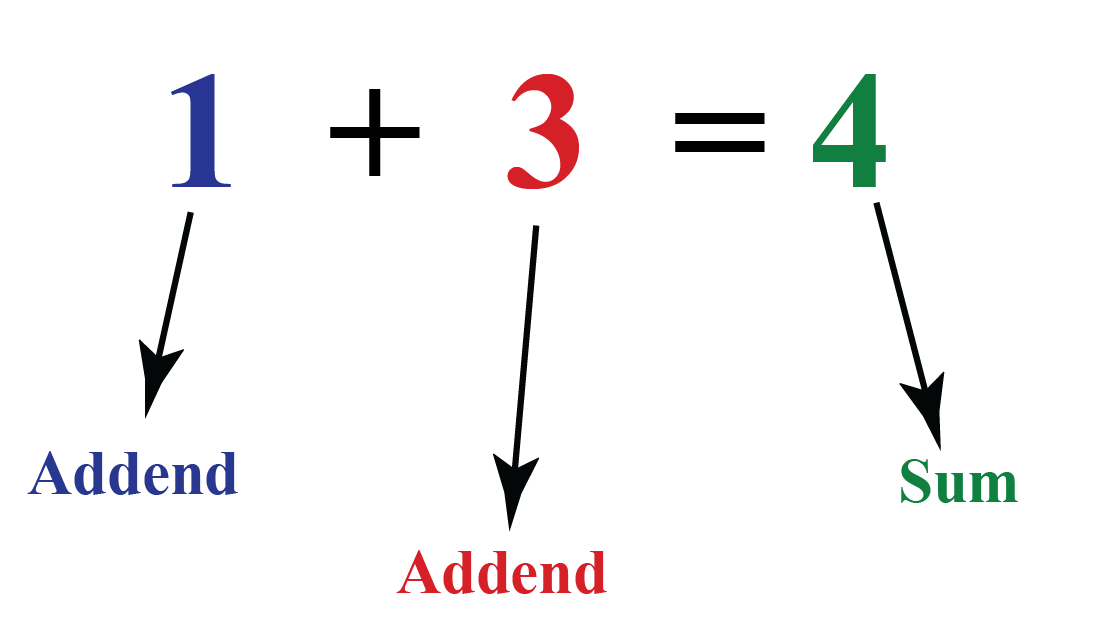## What is Sum?

### Sum: Definition

Sum is a way of putting things together.

The sum brings two or more numbers together to make a new total.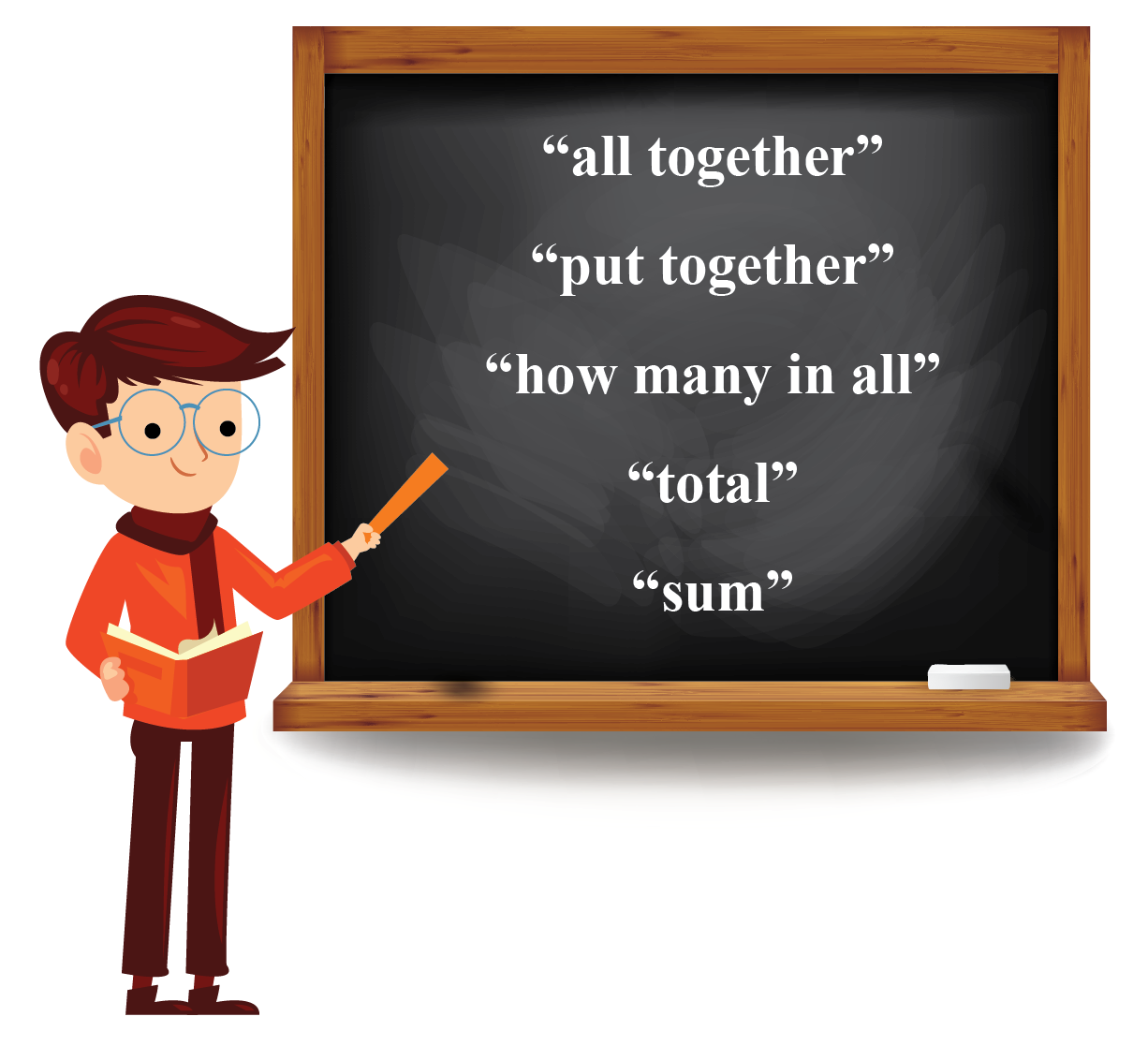The terms such as "all together, "put together," "how many in all," "total," and "sum"  indicates that you will need to add two or more numbers.

The plus sign (+) is used when two things are added together.

## What is the Sum of Two Numbers?

The sum of two numbers is just putting two numbers together.

Finding the sum of small numbers is not hard.

Let's look at an example to understand how to add numbers using our fingers.

Alaya is standing near a traffic signal.

She is trying to count the number of vehicles that have stopped at the red light signal.

She sees three buses at the signal.

She counts them by opening three fingers of one hand.Next, she sees two more buses that have stopped at the signal.

She counts them by opening two more fingers.Now, Alaya counts all the fingers opened in her hand to count the number of buses at the signal.

There are five open fingers which means that there are 5 buses at the signal.

Now, let's see how to find a sum using a number line.

We will find the sum of 3 and 2 using a number line.

First, draw a number line and encircle the first addend 3 on it.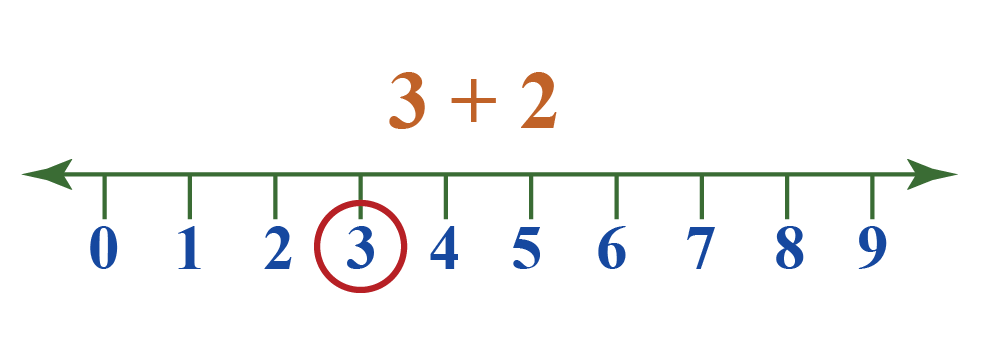Now, make two jumps to the right of 3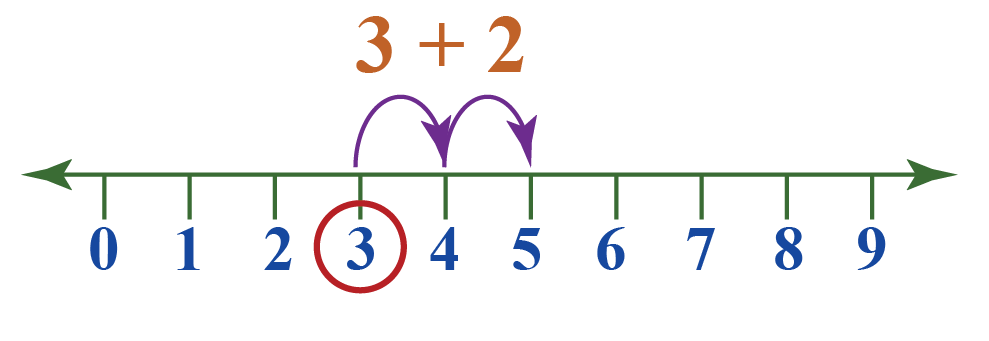The number you reach is 5

This is the sum.

### Sum: Calculator

Do you want to view more examples on how to add numbers?

You can explore this simulation to find the sum of two numbers.

Drag the points on the red line and blue line to change the number of candies.

You can observe that as the number of candies change, the sum of them changes too.

### Fun Facts

Do you know that two numbers and their sum form a "fact family"?

For example, the numbers 4, 5, and 9 form a fact family.

This is because $$4 + 5 = 9$$; $$5 + 4 = 9$$; $$9 - 4 = 5$$; and $$9 - 5 = 4$$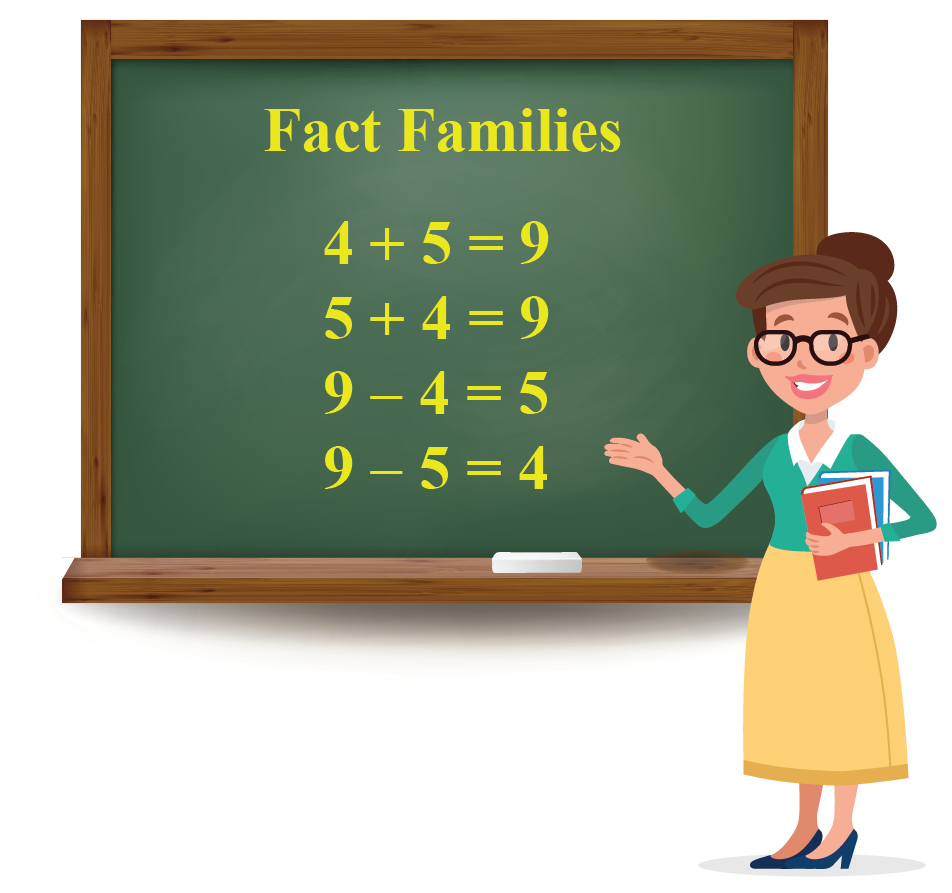So, now you know how the numbers interact with each other.

The sum of any two natural numbers is greater than either of them.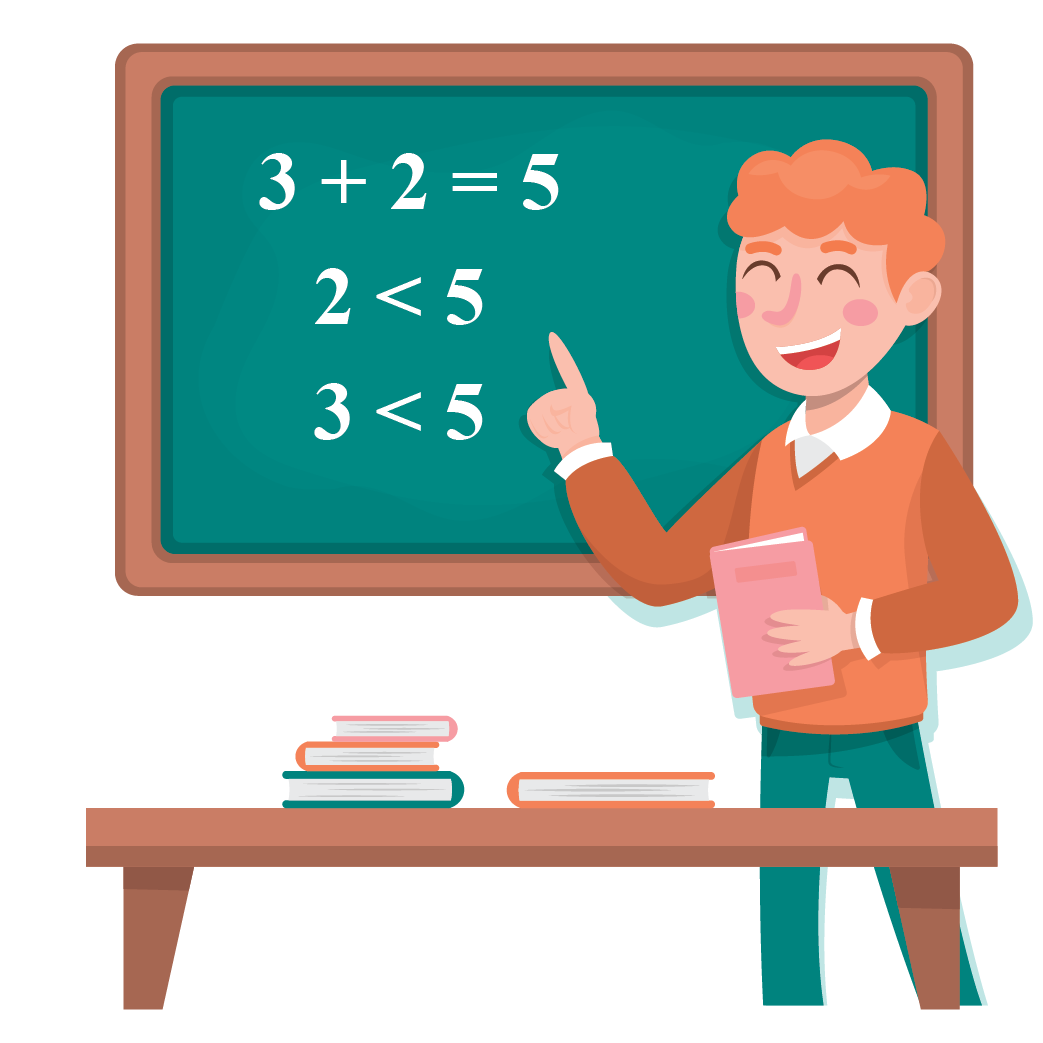More Important Topics
More Important Topics
Learn from the best math teachers and top your exams

• Live one on one classroom and doubt clearing
• Practice worksheets in and after class for conceptual clarity
• Personalized curriculum to keep up with school